Courses

# NEET Previous Year Questions (2014-20): Oscillations Class 11 Notes | EduRev

## Class 11 : NEET Previous Year Questions (2014-20): Oscillations Class 11 Notes | EduRev

The document NEET Previous Year Questions (2014-20): Oscillations Class 11 Notes | EduRev is a part of the Class 11 Course Physics Class 11.
All you need of Class 11 at this link: Class 11

Q 1. Average velocity of a particle executing SHM in one complete vibration is:    
A: Aω/2
B: Aω
C: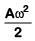D: Zero
Ans:
D
Solution:
In one complete vibration, displacement is zero. So, average velocity in one complete vibration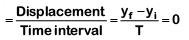Q 2. The displacement of a particle executing simple harmonic motion is given by y = A0+ Asinωt + Bcosωt
Then the amplitude of its oscillation is given by :    
A: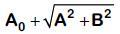B: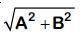C: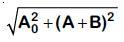D: A + B
Ans:
B
Solution: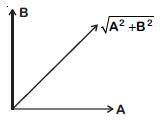y = A0+ Asinωt + Bsinωt
Equate SHM
y' = y – A0 = Asinωt + Bcosωt
Resultant amplitude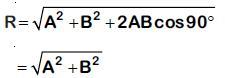Q 3. The radius of circle, the period of revolution, initial position and sense of revolution are indicated in the fig.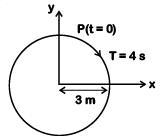y - projection of the radius vector of rotating particle P is:    
A: y(t) = –3 cos2πt, where y in m
B: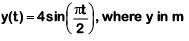C: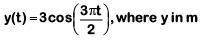D: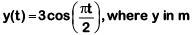Ans:
D
Solution:

At t = 0, y displacement is maximum, so equation will be cosine function.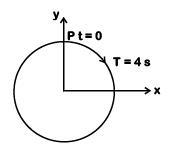T = 4s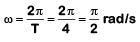y = a cosωt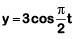Q 4. A pendulum is hung from the roof of a sufficiently high building and is moving freely to and fro like a simple harmonic oscillator. The acceleration of the bob of the pendulum is 20 m/s2 at a distance of 5 m from the mean position. The time period of oscillation is:-    
A: 2πs
B: πs
C: 2s
D: 1s
Ans:
B
Solution: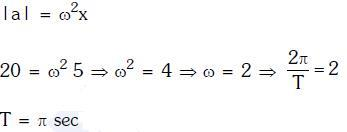Q 5. A particle executes linear simple harmonic motion with an amplitude of 3 cm. When the particle is at 2 cm from the mean position, the magnitude of its velocity is equal to that of its acceleration. Then its time period in seconds is:-    
A: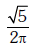B: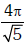C: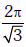D: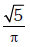Ans:
B
Solution: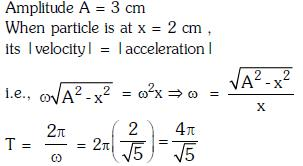Q 6. A particle is executing SHM along a straight line. Its velocities at distances x1 and x2 from the mean position are V1 and V2, respectively. Its time period is:    
A: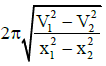B: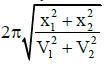C: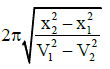D: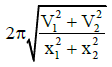Ans: C
Solution: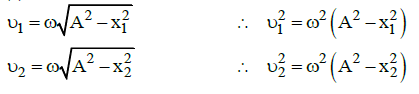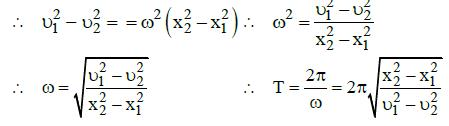Q 7. When two displacements represented by y1 = a sin(ωt) and y2 = b cos(ωt) are superimposed the motion is:    
A: simple harmonic with amplitude (a+b)/2
B: not a simple harmonic
C: simple harmonic with amplitude a/b
D: simple harmonic with amplitude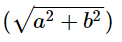Ans:
D
Solution: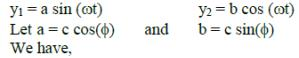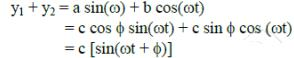where  c2 = a2 + b2  [since  a+ b2 = c2 cos (φ) + csin (φ) = c2 ]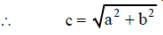The superimposed motion is simple harmonic with amplitude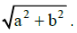Q 8. The oscillation of a body on a smooth horizontal surface is represented by the equation,
X = A cos (ωt)
Where X = displacement at time t
ω = frequency of oscillation
Which one of the following graphs shows correctly the variation ‘a’ with ‘t’?    
A: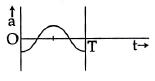B: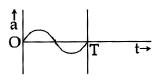C: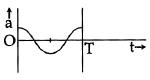D: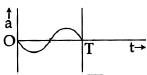Here, a = acceleration at time ‘t’ and T = time period
Ans:
A
Solution: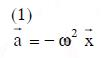Offer running on EduRev: Apply code STAYHOME200 to get INR 200 off on our premium plan EduRev Infinity!

## Physics Class 11

75 videos|312 docs|152 tests

,

,

,

,

,

,

,

,

,

,

,

,

,

,

,

,

,

,

,

,

,

;